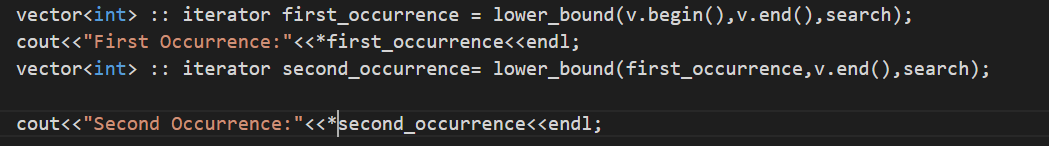← Back to all posts
##### Second Occurrence of an element

I tried finding the first occurrence of the element and then using that as a reference to find the next occurrence, but the output of the first and second is always the samehotnewtop
Geocube101 (645)

Assuming C++

``````// Vector to use
vector<int> nums = {1, 8, 7, 3, 6, 1, 2, 7, 5};
int target = 1;

// Get first occurence
vector<int>::iterator first_occurence = find(nums.begin(), nums.end(), target);

// Get second occurence using first occurence
vector<int>::iterator second_occurence = find(first_occurence + 1, nums.end(), target);

// Convert second occurrence iteratator to vector index
int index = second_occurence - nums.begin();
cout << index << ", " << nums[index] << endl;``````